Function Repository Resource:

# ASSTriangles

Create a list of all triangles with side lengths a and b and angle β opposite the side with length b

Contributed by: Ted Ersek
 ResourceFunction["ASSTriangles"][β,a,b] returns a list of all triangles with sides of lengths a and b and angle β opposite the side with length b.

## Details

ASSTriangles are known as angle-side-side triangles or side-side-angle triangles.
ResourceFunction["ASSTriangles"][β,a,b] assumes angle β is in radians.
ASSTriangles returns either a list of two triangles, a list of one triangle, or an empty list.
When ASSTriangles returns a non-empty list the elements have the head.
When ASSTriangles returns an empty list there are no triangles with the specified values for angle, side, side where the second side is opposite the specified angle.
Triangles returned by ASSTriangles have angle β at the origin and the side with length = a along the positive horizontal axis.ASSTriangles allows angle β strictly between 0 and π and lengths a, b to be any positive number.

## Examples

### Basic Examples (5)

There are two triangles with the specified angle, side, side for this example:

 In:=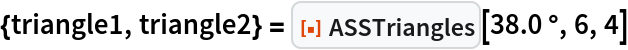Out=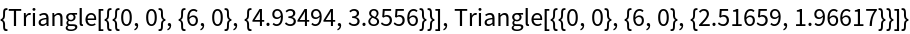The triangles above are the black outline and the gray triangle below:

 In:=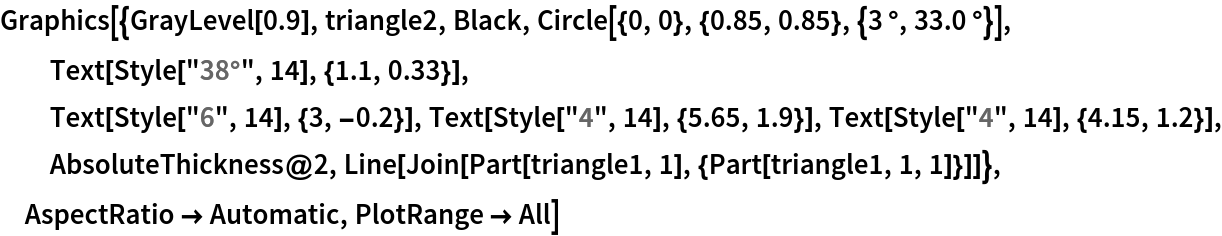Out=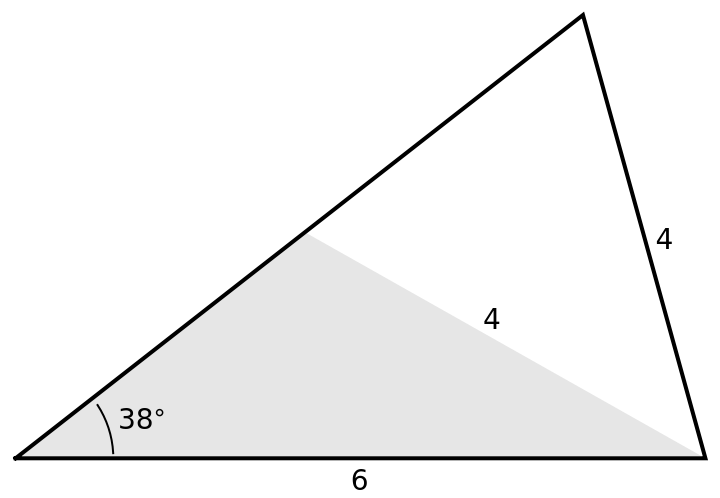The next two cells verify that both triangles above have the specified angle, side, side:

 In:=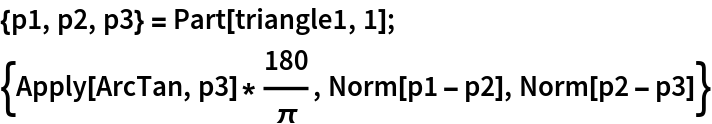Out=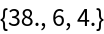In:=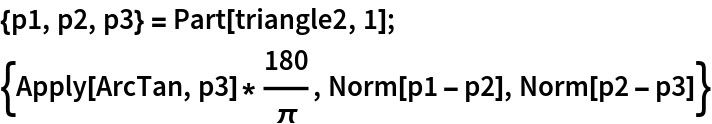Out=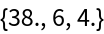Only one triangle has the specified angle side, side in this example:

 In:=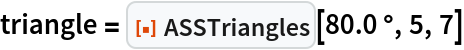Out=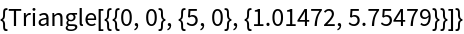In:=Out=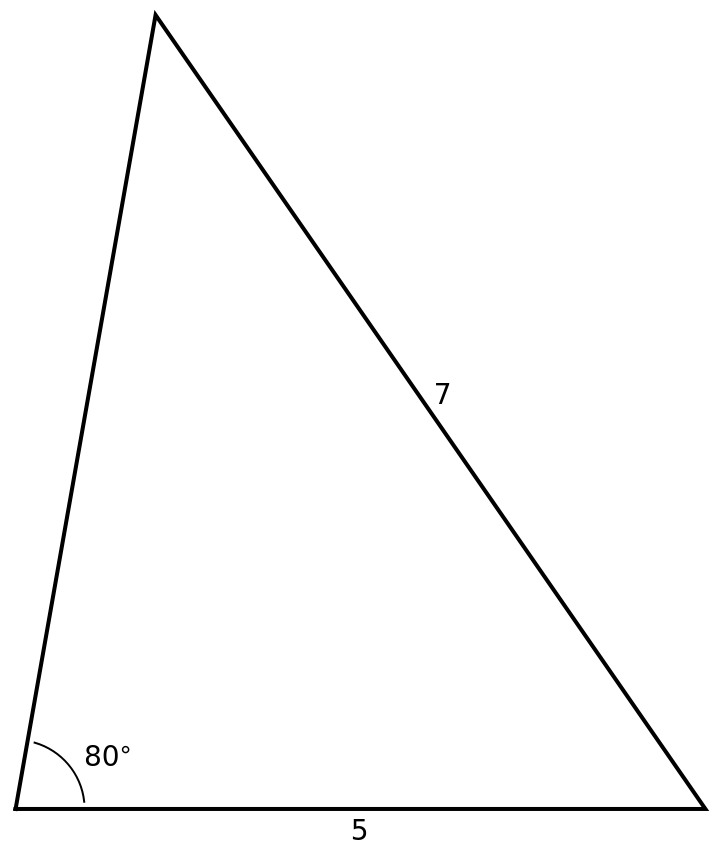The next cell verifies the triangle above has the specified angle, side, side:

 In:=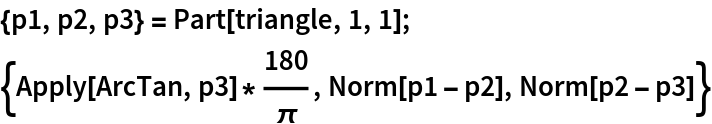Out=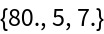ASSTriangles always returns one obtuse triangle when the specified angle is 90° or greater and the third argument is greater than the second argument. An example is in the next cell:

 In:=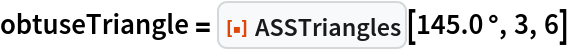Out=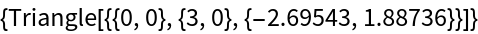In:=Out=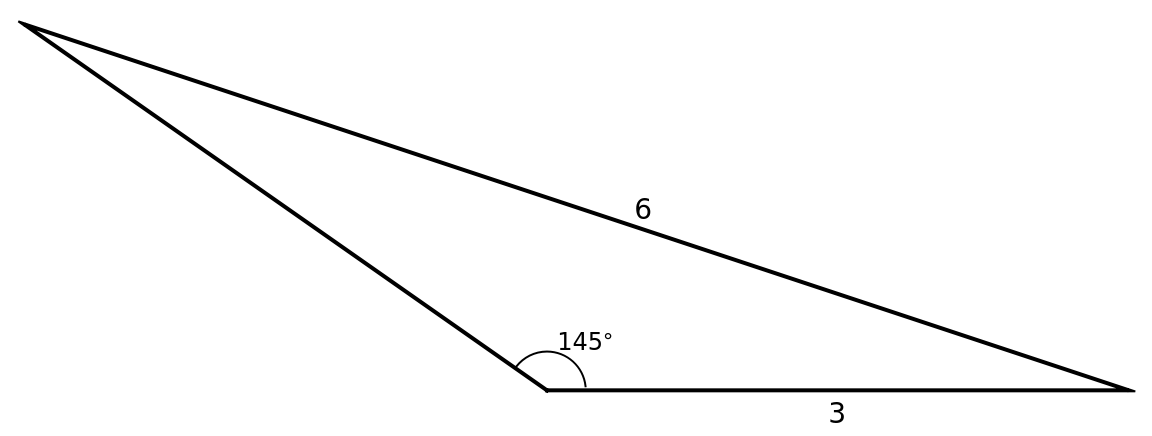The next cell verifies the triangle above has the specified angle, side, side:

 In:=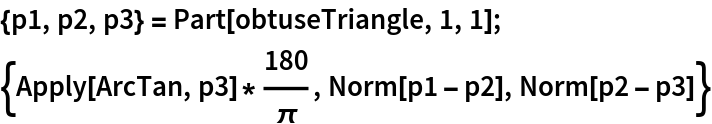Out=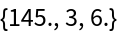The next example makes a (3,4,5) right triangle:

 In:=Out=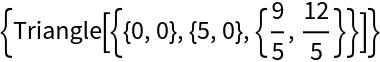In:=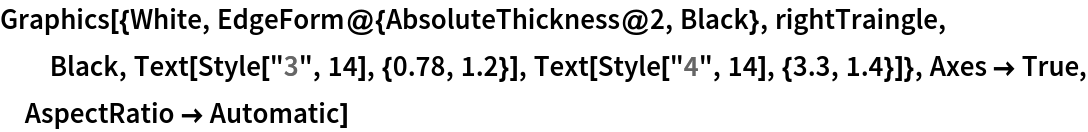Out=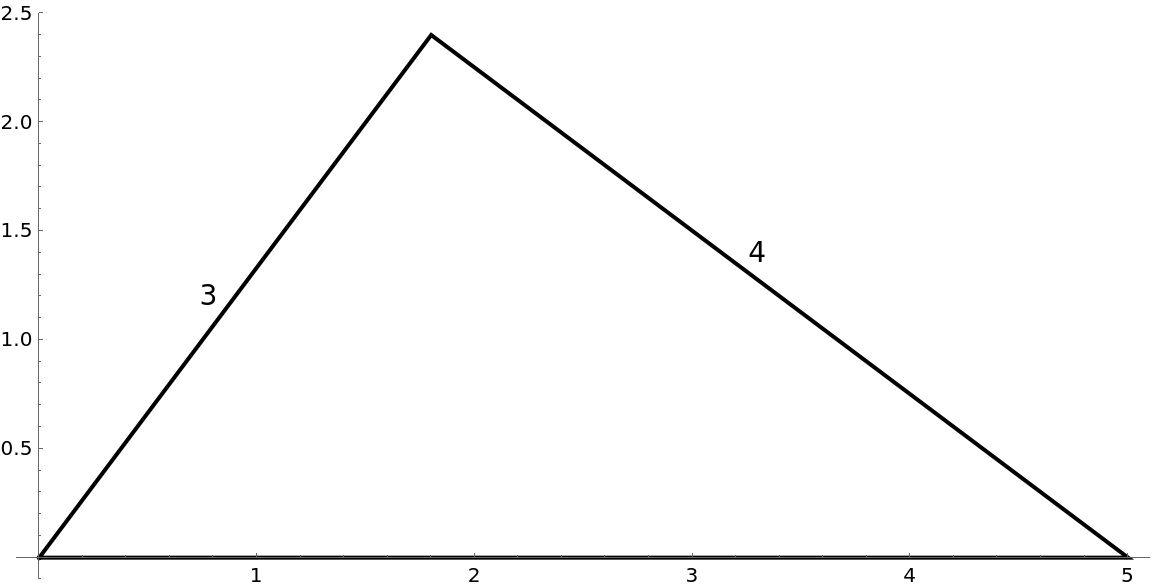The next cell verifies the triangle above has the specified angle, side, side:

 In:=Out=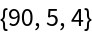The next example is a case where no triangle has the specified angle, side, side:

 In:=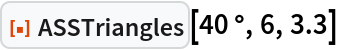Out=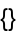In this example we would have a right triangle if the last argument of ASSTriangles was 6 Sin[40°]≈3.85673. The next graphics illustrates why no triangle has the angle, side, side specified in the last example:

 In:=Out=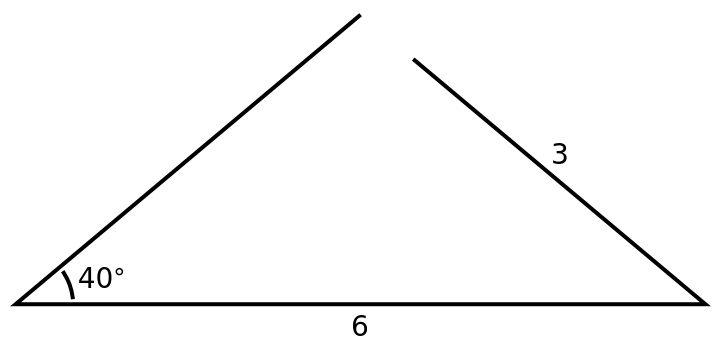### Scope (4)

When ASSTriangles is given exact input it returns exact results:

 In:=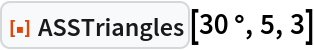Out=When ASSTriangles is given values with many digits of precision it returns results with all digits that can be be justified:

 In:=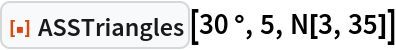Out=When one or more argument is symbolic and non-numeric ASSTriangles does nothing to change the input:

 In:=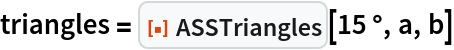Out=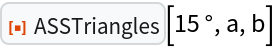We can use replacement rules to assign specific values for a, b in triangles above:

 In:=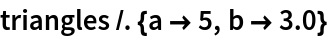Out=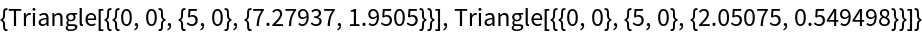We can also use Blockto assign values to a, b in triangles above:

 In:=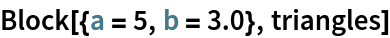Out=ASSTriangles displays an error message and returns the original input when given incorrect:

 In:=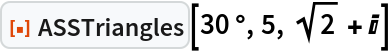Out=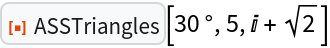No message is displayed in the next example because it would give a list of Triangles if b had an appropriate value:

 In:=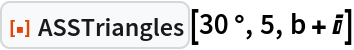Out=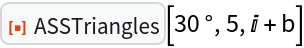### Neat Examples (1)

The next example allows you to see the result of providing ASSTriangles any values for three arguments:

 In:=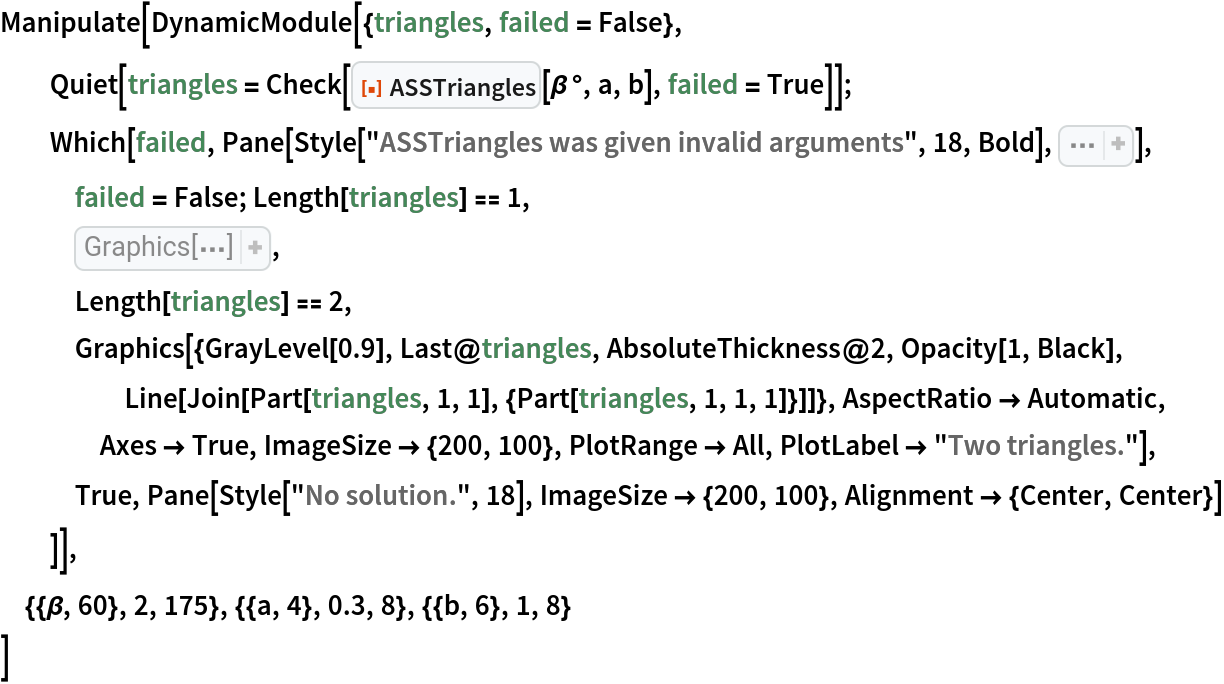Out=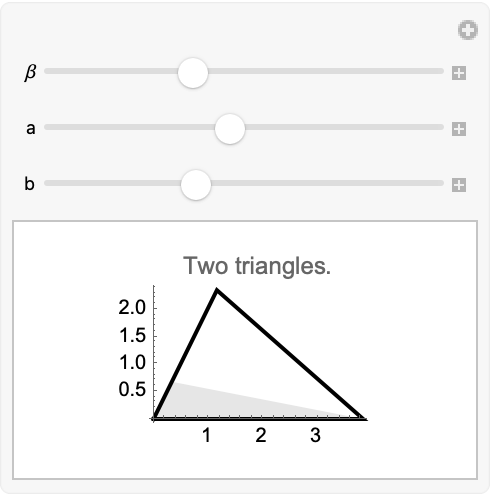Ted Ersek

## Version History

• 1.0.0 – 04 November 2022